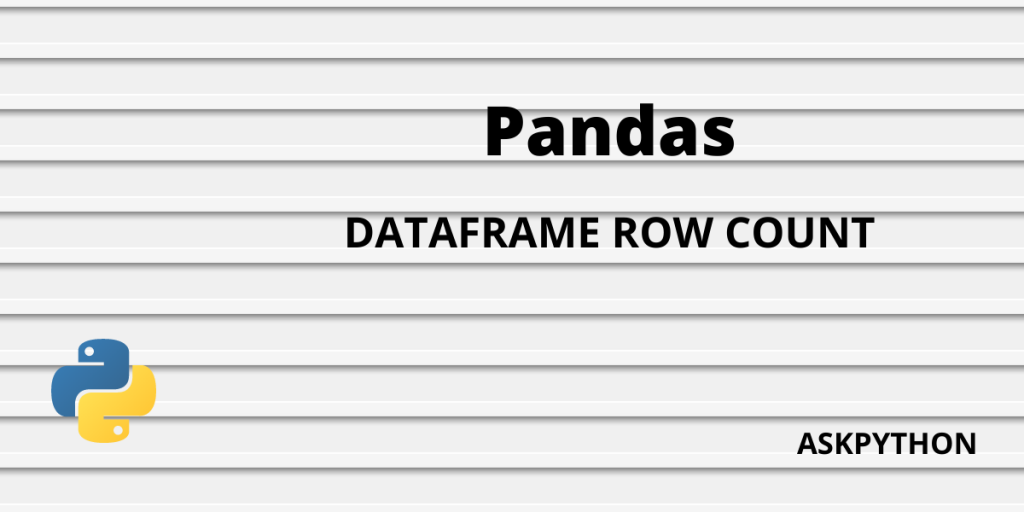# 6 Ways to Count Pandas Dataframe RowsWant to learn how to count Pandas data frame rows? In this article, we’ll learn how to do that with easy methods. Pandas is a Python library made for manipulating data in tables and data frames easily. Pandas have lots of systems functions, and in this article, we will be particularly focussing on those functions that help us derive row count for our data frames.

Let’s first start by creating a data frame.

```# Import pandas library
import pandas as pd

# initialize the variable data with your items
cars = [['Honda', 6], ['Hyundai', 5], ['Tata', 5.5]]

# Create the pandas DataFrame
cars_df = pd.DataFrame(cars, columns = ['Brand', 'Price'])

# print dataframe.
print(cars)
```

## Methods to Find Row Count of a Pandas Dataframe

There are primarily four pandas functions to find the row count of a data frame. We will discuss all four – their properties, syntax, function calls, and time complexities.

### Method 1: len(df.index)

Code:

```import pandas as pd

cars = [['Honda', 6], ['Hyundai', 5], ['Tata', 5.5]]

cars_df = pd.DataFrame(cars, columns = ['Brand', 'Price'])

# counting rows
print(len(cars_df.index))
```

The above code will return the number of rows present in the data frame, (3, in the example above). The syntax, len(df.index), is used for large databases as it returns only the row count of the data frame, and it is the fastest function that returns elements inside a data frame. Though being a lot similar by properties, it is faster than len(df) (method 4), as it has one less function call to execute.

### Method 2: df.shape[]

Code:

```import pandas as pd

cars = [['Honda', 6], ['Hyundai', 5], ['Tata', 5.5]]

cars_df = pd.DataFrame(cars, columns = ['Brand', 'Price'])

# counting rows
print(cars_df.shape)
```

This function is used to count rows and columns in a data frame, and the syntax df.shape returns both the row and column count of the tuple.

The [ ] brackets are used to denote the index, i.e. df.shape returns row count, and df.shape returns column counts. In time comparison it is slower than (df.index). ‘timeit’ testing shows that it is 3times much slower than len(df.index).

### Method 3: df[df.column].count()

Code:

```import pandas as pd

cars = [['Honda', 6], ['Hyundai', 5], ['Tata', 5.5]]

cars_df = pd.DataFrame(cars, columns = ['Brand', 'Price'])

# counting rows
print(cars_df[cars_df.columns].count())
```

This pandas function counts all the nonempty rows in the first column of a data frame. The time complexity increases with an increase in the number of rows. In the chart below, you can see that the time complexity is rather constant till the first 10,000 rows, but then start to increase after that. The drawback of this function is that it only counts the nonempty rows and leaves out the null ones.

### Method 4: len(df)

Code:

```import pandas as pd

cars = [['Honda', 6], ['Hyundai', 5], ['Tata', 5.5]]

cars_df = pd.DataFrame(cars, columns = ['Brand', 'Price'])

# counting rows
print(len(cars_df))
```

This function counts the length of the index, which is similar to the function len(df.index), but a little slower. If we go precisely by the time taken per loop, we find that len(df) is approximately 200ns slower than len(df.index). This difference can seem small but can cause major time differences when large data frames are used.

### Method 5: df.count()

This pandas function gives the count of the whole table, similar to df.shape[] function, but with some changes in readability. This function cannot be evoked to return the count of rows in a single column, instead, it returns the result in a tablet structure.

Code:

```import pandas as pd

cars = [['Honda', 6], ['Hyundai', 5], ['Tata', 5.5]]

cars_df = pd.DataFrame(cars, columns = ['Brand', 'Price'])

# counting rows and columns
print(cars_df.count())
```

Output:

Time complexity

## Method 6: df.[cols].count()

If we want the count of our data frame, specifically column-wise, then there are some changes in df.count() syntax which we have to make. The df.[col].count() syntax is what we need to mention to the compiler. This syntax counts the elements in a row, column-specific-wise.

This syntax is rather helpful when working with .csv files, which have a huge number of columns in them. This syntax also gives the count of empty rows in a column, which makes it more feasible.

Code:

```# Import pandas library
import numpy as np
import pandas as pd

# initialize the variable data with your items
cars = [['Honda', 6], [np.nan, np.nan], ['Hyundai', 5], ['Tata', 5.5]]

# Create the pandas DataFrame
cars_df = pd.DataFrame(cars, columns = ['Brand', 'Price'])

# counting column-specific row count
print(cars_df['Brand'].count())
```

Output:

## Conclusion

In this article, we have learned about different types of syntax and modules to count rows of a data frame. We learned how those syntaxes can be implemented in a program, and observed their time complexities as well. There are outputs as well to give you a better understanding, of what kind of results can you expect with different programs and syntaxes.

Hope this article helped you grasp a better understanding of the concepts of the data frame and row counts.